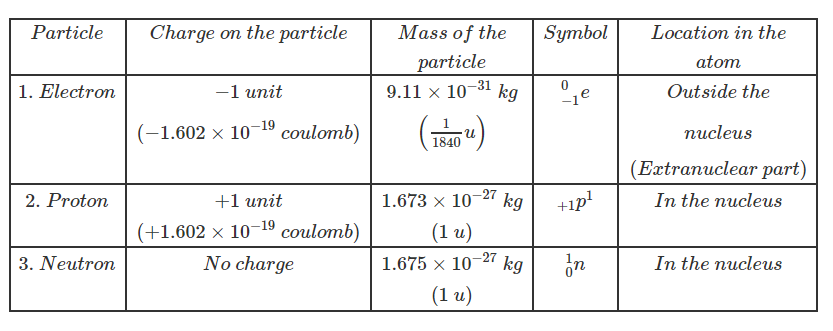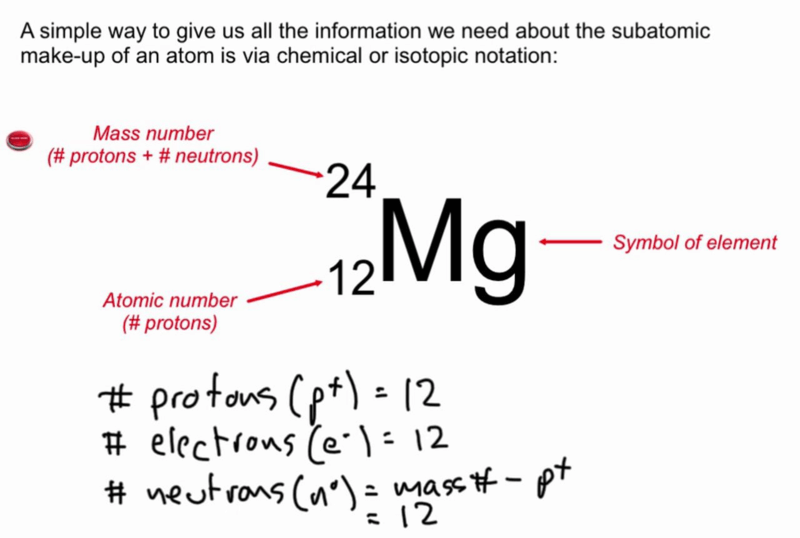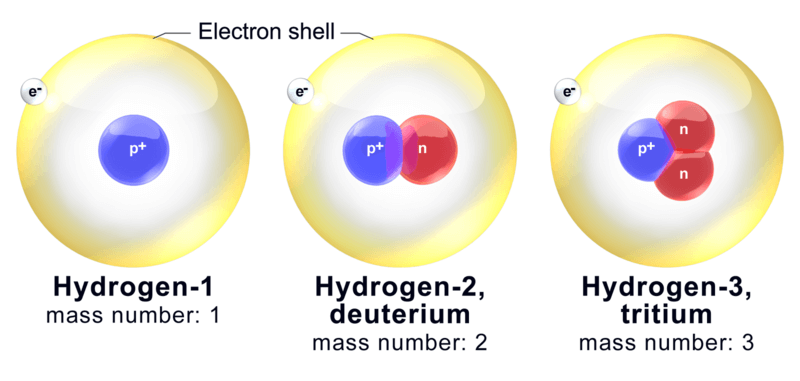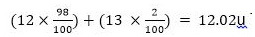# Structure Of The Atom Class 9 CBSE Notes - Chapter 4

Essentially, the structure of an atom comprises protons, neutrons and electrons. These basic components provide the mass and charge of the atoms. The nucleus comprises proton and neutron, with the electron orbiting around that.

## Introduction to Structure of an Atom

### Atoms

Atoms are the building blocks of matter. It is the smallest unit of matter that is composed of three sub-atomic particles: the proton, the neutron and the electron.

To know more about Atomic Structure, visit here.

### Cathode ray experiment

• J. J. Thomson discovered the existence of electrons.
• He did this using a cathode ray tube, which is a vacuum-sealed tube with a cathode and anode on one end that created a beam of electrons travelling towards the other end of the tube.
• The air inside the chamber is subjected to high voltage and electricity flows through the air from the negative electrode to the positive electrode.
• The characteristics of cathode rays (electrons) do not depend upon the material of electrodes and the nature of the gas present in the cathode ray tube.
• The experiment showed that the atom was not a simple, indivisible particle and contained at least one subatomic particle – the electron.

To know more about Cathode Ray Experiment, visit here.

### Electrons

• Electrons are the negatively charged sub-atomic particles of an atom.
• The mass of an electron is considered to be negligible, and its charge is -1.
• The symbol for an electron is e
• Electrons are extremely small.
• They are found outside the nucleus.

### Thomson’s model of an atom

• According to Thomson,(i) An atom consists of a positively charged sphere and the electrons are embedded in it. (ii) The negative and positive charges are equal in magnitude. So, the atom as a whole is electrically neutral
• The first model of an atom to be put forward and taken into consideration.
• He proposed a model of the atom be similar to that of a Christmas pudding/watermelon.
• The red edible part of the watermelon is compared with the positive charge in the atom.
• The black seeds in the watermelon are compared with the electrons which are embedded on it.

To know more about Thomson’s Model, visit here.

• Radioactivity is the term for the process by which an unstable nucleus of an atom loses energy by giving out particles.
• It does so by giving out particles such as alpha and beta particles.
• This process is spontaneous.
• An atom is unstable if the nucleus has an imbalance, meaning a difference in the protons and neutrons.

## Rutherford Model

### Rutherford’s experiment and observations

In this experiment, fast-moving alpha (α)-particles were made to fall on a thin gold foil. His observations were:

• A major fraction of the α-particles bombarded towards the gold sheet passed through it without any deflection, and hence most of the space in an atom is empty.
• Some of the α-particles were deflected by the gold sheet by very small angles, and hence the positive charge in an atom is not uniformly distributed.
• The positive charge in an atom is concentrated in a very small volume.
• Very few of the α-particles were deflected back, that is only a few α-particles had nearly 180o angle of deflection. So the volume occupied by the positively charged particles in an atom is very small as compared to the total volume of an atom.

#### For More Information On The Gold Foil Experiment, Watch The Below Video:### Rutherford’s model of an atom

Rutherford concluded the model of the atom from the α-particle scattering experiment as:

(i) There is a positively charged centre in an atom called the nucleus. Nearly all the mass of an atom resides in the nucleus.

(ii) The electrons revolve around the nucleus in well-defined orbits.

(iii) The size of the nucleus is very small as compared to the size of the atom.

### Drawbacks of Rutherford’s model

• He explained that the electrons in an atom revolve around the nucleus in well-defined orbits. Particles in a circular orbit would experience acceleration.
• Thus, the revolving electron would lose energy and finally fall into the nucleus.
• But this cannot take place as the atom would be unstable and matter would not exist in the form we know.

## Be More Curious!!!

• The Millikan’s Oil Drop Experiment was an experiment performed by Robert A. Millikan and Harvey Fletcher in 1909 to measure the charge of an electron.
• In the experiment, Millikan allowed charged tiny oil droplets to pass through a hole into an electric field.
• By varying the strength of electric field, the charge over an oil droplet was calculated, which always came as an integral value of ‘e.’
• The conclusion of this is that the charge is said to be quantized, i.e. the charge on any particle will always be an integral multiple of e which is 1.6*10-19

To know more about Rutherford Model, visit here.

## Neil Bohr Model

### Properties of electrons, protons and neutronsBohr’s Model of an atom

Bohr came up with these postulates to overcome the objections raised against Rutherford’s model:

• Electrons revolve around the nucleus in stable orbits without emission of radiant energy. Each orbit has a definite energy and is called an energy shell or energy level.
• An orbit or energy level is designated as K, L, M, N shells. When the electron is in the lowest energy level, it is said to be in the ground state.
• An electron emits or absorbs energy when it jumps from one orbit or energy level to another.
• When it jumps from a higher energy level to lower energy level, it emits energy while it absorbs energy when it jumps from lower energy level to higher energy level.

To know more about Bohr Model, visit here.

### Orbits

Orbits are energy shells surrounding the nucleus in which electrons revolve.

### Electron distribution in different orbits

The distribution was suggested by Bohr and Bury;

• The maximum number of electrons present in a shell is given by the formula 2n2, where ‘n’ is the orbit number or energy level index, 1,2,3,….
• The maximum number of electrons in different shells are as follows: the first orbit will have 2*12=2, the second orbit will have 2*2Msup>2=8, the third orbit will have 2*32=18, fourth orbit 2*42=32 and so on.
• The shells are always filled in a step-wise manner from the lower to higher energy levels. Electrons are not filled in the next shell unless previous shells are filled.

### Valency

• The electrons present in the outermost shell of an atom are known as the valence electrons.
• The combining capacity of the atoms or their tendency to react and form molecules with atoms of the same or different elements is known as valency of the atom.
• Atoms of elements, having a completely filled outermost shell show little chemical activity.
• Their combining capacity or valency is zero.
• For example, we know that the number of electrons in the outermost shell of hydrogen is 1, and in magnesium, it is 2.
• Therefore, the valency of hydrogen is 1 as it can easily lose 1 electron and become stable.
• On the other hand, that of magnesium is 2 as it can lose 2 electrons easily and also attain stability.

To know more about Valency, visit here.

### Atomic Number

The number of protons found in the nucleus of an atom is termed as the atomic number. It is denoted by the letter ‘Z’.

### Mass number and representation of an atom

Protons and neutrons are present in the nucleus, so the mass number is the total of these protons and neutrons.To know more about Atomic Number and Mass number, visit here.

### Isotopes and Isobars

Isotopes are defined as the atoms of the same element, having the same atomic number ( number of protons ) but different mass numbers ( number of protons+neutrons ).
For example: In the case of Hydrogen we have:Atoms of different elements with different atomic numbers, which have the same mass number, are known as isobars.
For example, Calcium and Argon: both have the same mass number – 40
20Ca40 and 18Ar40

To know more about Isotopes and Isobars, visit here.

### Calculation of mass number for isotopic elements

When an element has an isotope, the mass number can be calculated by the different proportions it exists in.

For example take 98% Carbon-12u and 2% Carbon-13uThis does not mean that any Carbon atoms exists with the mass number of 12.02u. If you take a certain amount of Carbon, it will contain both isotopes of Carbon, and the average mass is 12.02 u.

To discover more questions or relevant concepts on Structure Of Atom Class 9 Notes, register at BYJU’S.